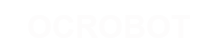#### Site Tools

en:reference:language:assignment_operator

# = assignment operator (single equal sign)

Stores the value to the right of the equal sign in the variable to the left of the equal sign.

The single equal sign in the C programming language is called the assignment operator. It has a different meaning than in algebra class where it indicated an equation or equality. The assignment operator tells the microcontroller to evaluate whatever value or expression is on the right side of the equal sign, and store it in the variable to the left of the equal sign.

## Example

``` int sensVal;                 // declare an integer variable named sensVal
senVal = analogRead(0);       // store the (digitized) input voltage at analog pin 0 in SensVal```

## Programming Tips

The variable on the left side of the assignment operator ( = sign ) needs to be able to hold the value stored in it. If it is not large enough to hold a value, the value stored in the variable will be incorrect.

Don't confuse the assignment operator [ = ] (single equal sign) with the comparison operator [ == ] (double equal signs), which evaluates whether two expressions are equal.